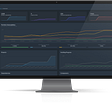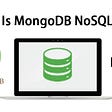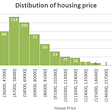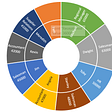# DAX Series: 3. Measures vs Calculated Columns in DAX

(You can also find the article on my website together with other articles and more interesting things)

This is the third article of the series: DAX Series — Learn the core of Power BI
3. Measures vs Calculated Columns

In the end of this article I have a link to my GitHub where you can find one empty Power BI Model and one full model with the calculation that I have done in this article.

In this article I will describe in detail the difference between Measures and Calculated Columns in DAX. I will describe in which use cases each of these can be used and common pitfalls.

You can also find the story here:
https://eliasnordlinder.com/power-bi/f/dax-series-3-measures-vs-calculated-columns-in-dax

# What are Calculated Columns?

I will begin this article by showing an example of a calculated columns and then describe its qualities later. The easiest way to add a calculated column is by going to the “Data” view in Power BI, go to the home ribbon and click “New Column”, see picture below:

When pressing the New Column you will be able to write the calculation for your calculated column in the formula bar.
Below are the calculation for “Total Sales Calc Col” in my example.

After you have created the calculated column it is now added to the existing table where you created the column. In my example I created it in the sales table and is therefore added as a column in the sales table.

What is really going on behind the scenes to calculate the calculated column in my example?

If you look closely at the first picture it says, “and calculates values for each row”. This is a very important concept and means that each value is calculated on row by row basis.

First, the order quantity is multiplied by the unit price times (1 minus the discount) for row 1. After that the quantity is multiplied by the unit price times (1 minus the discount) for row 2 and so on.

The second important concept to remember is that this calculation is computed once and will never be computed again afterwards.

“Calculated columns are computed once and stored in a table” Alberto Ferrari

Let me describe these concepts more and what consequences these have for the model.

## Good things about Calculated columns

• Easy to Understand

As the calculated columns are calculated “Row by Row” (In the Row Context) makes them easier to understand than measures, especially if you come from an Excel background.

Total Sales which I calculated earlier can be calculated with the same setup in Excel which is very similar to the Calculated Column.

It is easy to understand how calculated column works and how to use them, which makes the learning curve shorter for learning how to work with calculated columns. As they are stored in the table and you can see the calculated column physically also make it less abstract and easier to comprehend.

• Useful in slicers

One useful place for calculated columns is in slicers.
If you are using a small table (< 5 million rows) one can create a calculated columns for buckets for example. (For big tables a specific segmentation table is better, which I will describe in article 13. Dynamic Tables).

Example below:

You can divide the sales values in different buckets and then use as a slicer in your report.

Now that you have began to like calculated columns, let us go into why you should almost never use them, besides some few use cases when they are necessary, which I will describe later.

• Takes up space in RAM memory

The convenient things with that Calculated columns are stored in a table after they are calculated also leads to negative consequences. As they are stored they will make your data model bigger, and they will take up space in your RAM memory. More calculated columns will mean a bigger model and taking up more and more RAM memory.

If you are coming from a SQL background, columns are then calculated at query time and do not take up memory, but here they are computed during database processing and do take up memory.

If you get used to creating calculated columns, it might mean that you create new ones every time you want to create a new calculation. After a while you will have a lot of calculated columns and your model will take up a lot of memory.

## Possible to perform the same calculations without taking up RAM?

Now you might wonder what you should do instead. Calculated columns did solve most of the things you wanted to calculate, but now I am saying that you should not use them anymore. Is there anyway to perform all of these calculations but to not take up this precious RAM memory?

The answer to this is that there is, and the answer to this is Measures.

# What are Measures?

Measures are very well suited for calculating aggregates, such as sums or averages of specific columns.

Let us go through the creation of measures and then discuss what they are in more detail. The easiest way to create a measure is in the home ribbon on the “Report” page:

Once again you will have the formula bar where you can write the measure. Below is the how Total Sales are calculated as a measure instead as of a calculated column.

A measure is not stored in a table as a calculated column is. Therefore we need to include it in a visualization (Or use external tools as DAX Studio) to see how the measure looks.

Below I have inserted the measure together with the previously calculated calculated column and some other columns as a table visualization.

At this moment, it looks like a measure is just a more complicated way of calculating the total sales, but there are a lot more going on here. Let us discuss it the same way as for calculated columns.

• They are not stored in the RAM Memory.

As you saw above was measures not added as extra columns in tables when they are created. The reason for this is that Measures are not stored in RAM memory as calculated columns are. This is actually one of the greatest benefits of using measures. Measures are only calculated at query time, ”when they are used”, similar to computed columns in SQL.

If you can have a lot of measures in a Power BI Model without using up a lot of RAM Memory.

• Computing Ratio of Aggregates

Another example where measures must be used instead of calculated columns is when computing ratios of aggregates, such as percentages. Calculated columns can not compute this, while measures are a great tool to work with when working with aggregates and percentage.

This gives the following result

• Difficult to understand

Measures are more difficult to understand than calculated columns.
For me, it is especially two things that creates this confusion, they are not calculated on row-by-row basis and they are not stored physically in a table.
Let us discuss the measure for Total Sales earlier.

The first thing that you will notice if you compare it to the calculated column is SUMX. This is an iterator which I will describe more in detail in the next chapter. But to describe shortly, it does what the picture to the right says, it iterates through every single row in a specific table (Sales) and performs some logic/expression on this table.

If you try to write a measure like a calculated column you will get this error:

“A single value for ‘Order Quantity’ in the table ‘Sales’ cannot be determined. This can happen when a measure formula refers to a column that contains many values without specifying an aggregation such as min, max, count or sum.”

What does it mean that “A single value cannot be determined”?
Let us take help of the DAX gods again.
(I will not discuss the both parts of this error message provided by Microsoft actually is misleading and wrong in this case, but SQLBI discusses this more in their blog post)

The above error is the first thing that makes Measures more difficult to understand than calculated columns. Measures does not automatically operate in a “Row context” (Calculated row by row) such as calculated column does. Therefore you cannot write a measure like that, you must “Invoke a row context”, which is a short correct translation of the error message above.

To invoke a row context you can use an iterator such as SUMX in the example above. Let us go through that expression again:

What have happened now is that you have created a row context by using SUMX and provides a table, ‘Sales’, which the expression will iterate through row by row. In short one can say that you have a virtual table in the background, Sales, and then you create a virtual column for each row which is the expression above, sales amount in this case.

# Conclusion

• Calculated Columns takes up precious RAM memory and is stored physically in your model, while measures are calculated on query time and is not stored physically in your model.
• Always use Measures if you could express something both with Calculated Columns and Measures.
• Measures are more difficult to learn and comprehend, but the reward for learning them is really big.
• You cannot use the same syntax in Calculated Columns and Measures as Calculated Columns have a Row Context inherited while Measures does not. For example use SUMX to calculate the expression of two columns, DIVIDE for the division etc.

As this subject is so important there are a lot of additional resources about it as well, if you want to learn more about the difference between Calculated Columns and measures. I have posted some of the ones I really like below:

• Blue Granite has a blog post about the difference of Measures and Calculated Columns as well.
• Curbal has a great DAX friday video about the subject as well called “DAX Fridays! #46: What is the difference between calculated column and measure in Power BI?”
• EnterpriseDNA has one video about the differences in their ultimate beginners guide on youtube, called “Measures vs Calculated Columns — (1.5) Ultimate Beginners guide to DAX”.
• Radacad has written a blog post as well have a youtube video about the subject called “Measure vs Calculated Column in Power BI The Mysterious Question Not”
• SQLBI has written both a blog post about the subject and posted a youtube video discussing the differences, called “Calculated Columns and Measures in DAX”.

# References

EnterpriseDNA
Youtube Video: Measures vs Calculated Columns — (1.5) Ultimate Beginners guide to DAX

SQLBI
Youtube Video: Measures vs Calculated Columns
Blog Post: Calculated Columns and Measures in DAX

# Power BI Models available on GitHub

You can find the Power BI files for all of the Blog posts below:
https://github.com/EliasNo/DAX-Series

Inside PowerBI-Datamodels you can find the folder 3. Measures vs Calculated Columns, where I have posted the “Clean version” and the “Answer Key” with all the measures and similar done in this article.

Change the parameter below to where you save the excel sheet and you should be able to transform the data as well.

Thank you very much for reading my blog!

Drop a comment below about what you thought about the content, or if you want to mention how you use DAX in your daily work.

I would be interested to hear more about how other people see these things.

If you enjoy reading my stories, and would like to support me as a writer, consider to sign up to become a Medium member using this link, and I can earn a small commission, with no extra cost for you.

# Questions or inquiries

Email: Elias.Nordlinder@gmail.com
Webpage: eliasnordlinder.com

Have a great week everyone
//Elias

--

--

--

## More from Elias Nordlinder

Data Analyst at Visma. Previous BI Consult with MSc in Economics and Finance. Love problem solving/analytics and to teach data to other people.

Love podcasts or audiobooks? Learn on the go with our new app.

## Deploying Dependency Track as a Container in Azure and building a PipeLine with Azure Devops## How PMs can interview engineers## How Automated Web Scraping can Ease Your Pain## How to create a bootable flash drive with command prompt(cmd)## Jira Automation - Changing Status & Creating Clone & Adding Comment| Best Practices## Leetcode solution — 42. Trapping Rain Water## Elias Nordlinder

Data Analyst at Visma. Previous BI Consult with MSc in Economics and Finance. Love problem solving/analytics and to teach data to other people.

## EXPLORATORY DATA ANALYSIS AND VISUALIZATION IN EXCEL## Excel Charts for Data Analysis & Reporting## Google Data Analytics Course Capstone Project## Hotel Booking Analytics